# PyTorch深度学习

## 深度学习构建模块: Affine maps, non-linearities and objectives

### Affine Maps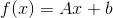Pytorch和大多数其他深度学习框架与传统的线性代数有所不同.它映射输入的是行而不是列. 也就是说, 下面的输出的第行是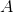的输入的第行加上偏置项的映射. 看下面的例子.

# 作者: Robert Guthrie

import torch
import torch.nn as nn
import torch.nn.functional as F
import torch.optim as optim

torch.manual_seed(1)

lin = nn.Linear(5, 3)  # 从 R^5 映射到 R^3, 参数 A, b
# 数据是 2x5\.  A 是从 5 映射到 3... 我们能在A下映射得到想要的数据吗?
print(lin(data))  # 是的


### Non-Linearities(非线性)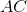是一个矩阵,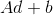是一个向量, 所以我们看到组合两个affine maps会得到一个affine map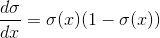# 在pytorch中, 很多的non-linearities是在torch中.是功能性的 (我们将它记为 F)
# 这意味着 non-linearites 不像 affine maps 一样拥有参数.也意味着它们再训练时没有可以更新的参数.
#
print(data)
print(F.relu(data))


### Softmax and Probabilities(Softmax和概率分布)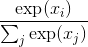# Softmax也在 torch.nn.functional
print(data)
print(F.softmax(data, dim=0))
print(F.softmax(data, dim=0).sum())  #总和为一因为他是概率分布!
print(F.log_softmax(data, dim=0))  # 他也是 log_softmax


### Objective Functions(目标函数)

Objective function 是一个目标函数，你训练网络的目的是使其最小(在这种情况下, 它通常被称为 损失函数成本函数 ). 首先选择一个训练实例, 通过神经网络运行它, 计算输出的损失. 然后利用损失函数的导数来更新模型的参数. 直观地说, 如果你的模型对答案完全有信心, 但答案是错误的, 你的损失就会很高. 如果它的答案非常有信心, 而且答案是正确的, 那么损失就会很低.

## Optimization and Training(优化和训练)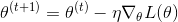## Creating Network Components in Pytorch(在Pytorch中创建神经元)

### 示例： Logistic Regression Bag-of-Words classifier(例子: 基于词袋表示法的逻辑斯蒂回归分类器)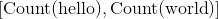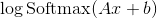data = [("me gusta comer en la cafeteria".split(), "SPANISH"),
("Give it to me".split(), "ENGLISH"),
("No creo que sea una buena idea".split(), "SPANISH"),
("No it is not a good idea to get lost at sea".split(), "ENGLISH")]

test_data = [("Yo creo que si".split(), "SPANISH"),
("it is lost on me".split(), "ENGLISH")]

# word_to_ix 将在词汇中的单词映射为一个特征数,
# 这个特征数就是单词在词袋中的索引
word_to_ix = {}
for sent, _ in data + test_data:
for word in sent:
if word not in word_to_ix:
word_to_ix[word] = len(word_to_ix)
print(word_to_ix)

VOCAB_SIZE = len(word_to_ix)
NUM_LABELS = 2

class BoWClassifier(nn.Module):  # 从 nn.Module继承!

def __init__(self, num_labels, vocab_size):
# 在 nn.Module中调用初始化函数. 不要被这个困惑,
# 这个做法经常在 nn.Module见到
super(BoWClassifier, self).__init__()

# 定义你需要的变量. 在本例中, 我们需要affine mapping的系数 A 和 b.
# Torch 定义了可提供 affine map的nn.Linear().
# 确定你理解了为什么输入矩阵的维度是 vocab_size而输出的是num_labels!
self.linear = nn.Linear(vocab_size, num_labels)

# 注意!  non-linearity log softmax 没有系数!
# 所以我们在这并不需要担心

def forward(self, bow_vec):
# 将输入引入到线性神经元层中, 随后引入到log_softmax.
# 在torch.nn.functional中有很多非线性和其他的函数
return F.log_softmax(self.linear(bow_vec), dim=1)

def make_bow_vector(sentence, word_to_ix):
vec = torch.zeros(len(word_to_ix))
for word in sentence:
vec[word_to_ix[word]] += 1
return vec.view(1, -1)

def make_target(label, label_to_ix):

model = BoWClassifier(NUM_LABELS, VOCAB_SIZE)

# model知道它的系数.第一个输出的是A, 第二个是b.
# 当你在模块__init__函数中指定一个神经元去分类变量, self.linear = nn.Linear(...)被执行
# 随后从Pytorch devs通过Python magic, 你的模块(在本例中, BoWClassifier) 将会存储 nn.Linear的系数
for param in model.parameters():
print(param)

sample = data
bow_vector = make_bow_vector(sample, word_to_ix)
print(log_probs)


label_to_ix = {"SPANISH": 0, "ENGLISH": 1}


# 在我们训练前运行训练集, 去看看前后的变化
for instance, label in test_data:
log_probs = model(bow_vec)
print(log_probs)

# 在矩阵中输出"creo"列
print(next(model.parameters())[:, word_to_ix["creo"]])

loss_function = nn.NLLLoss()
optimizer = optim.SGD(model.parameters(), lr=0.1)

# 通常你想要多次浏览训练集.100比起实际数据集是很多的, 但实际数据集会多于2个实例.
# 通常, 在5到30之间是合理的.

for epoch in range(100):
for instance, label in data:
# 步骤 1\. 牢记 Pytorch 会积累梯度.
# 我们需要在每一例前清理掉

# 步骤 2\. 制作我们的 BOW 向量 并且我们必须将目标封装在变量中并且为整数 .
# 例如, 如果目标是"西班牙语", 则封装为整数0.对于损失函数而言, 概率分布的
# 第0列对应的是"西班牙语"的损失函数.
#

# 步骤 3\. Run our forward pass.
log_probs = model(bow_vec)

# 步骤 4\. 计算损失, 梯度, 通过调用optimizer.step()来更新系数
#
loss = loss_function(log_probs, target)
loss.backward()
optimizer.step()

for instance, label in test_data:
log_probs = model(bow_vec)
print(log_probs)

# 对应于西班牙语上升, 英语下降!
print(next(model.parameters())[:, word_to_ix["creo"]])